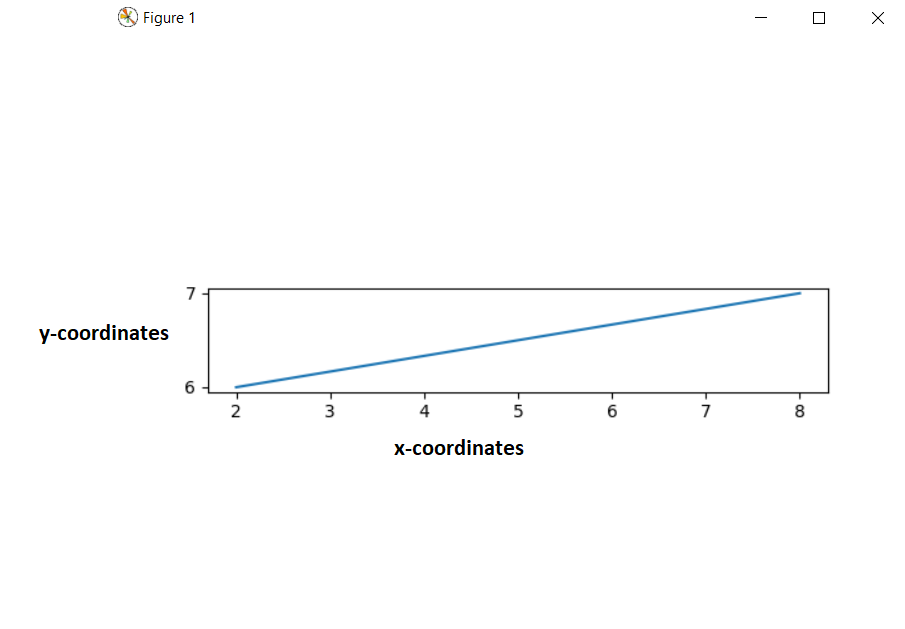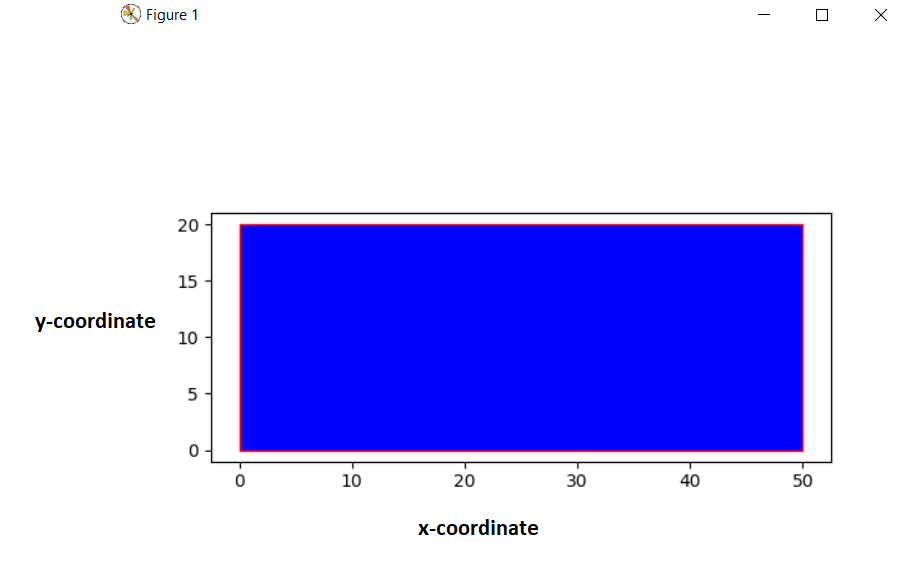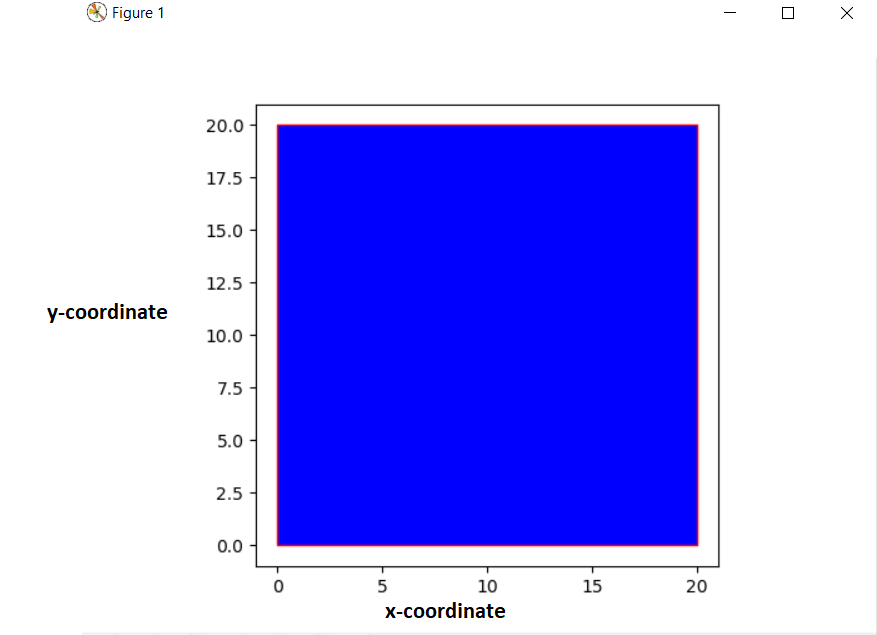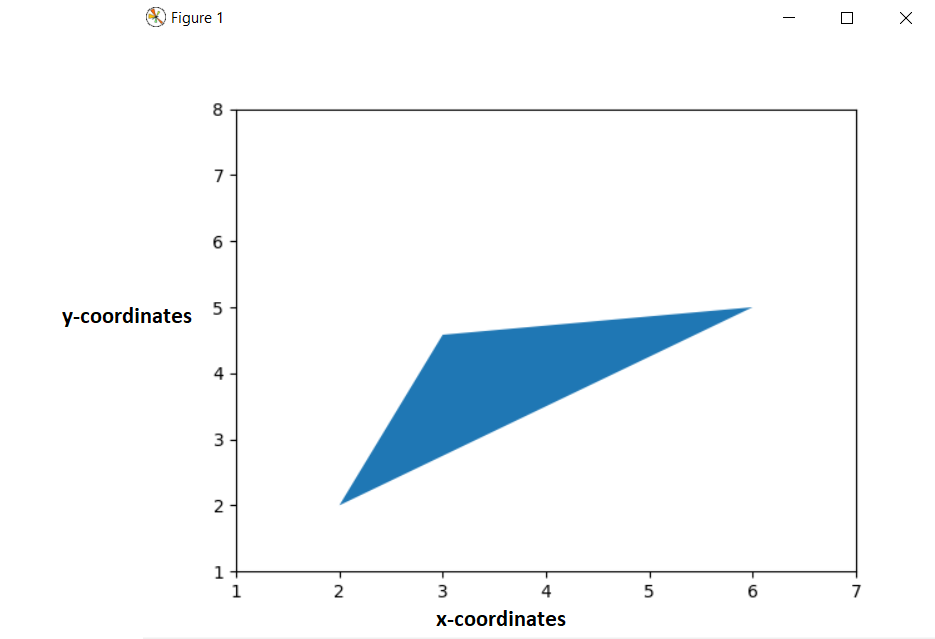# How to draw shapes in matplotlib with Python

In this article, we are going to learn how to draw different types of basic shapes like Lines, Rectangle, Square, Circle, Triangle in matplotlib using Python. For this, we need some basic concepts of two popular modules in the field of plotting figure i.e. Numpy and Matplotlib. In this article during drawing different types of shapes, we use the concept of “plt.gca()” which returns the current axes instances on the current figure.

## Drawing different types of shapes

Here, we are going to draw 5 basic shapes. Let’s start with Line:-

### Draw line shape in matplotlib – Python

```import matplotlib.pyplot as plt
plt.axes()
line = plt.Line2D((2, 8), (6, 7), lw=1.5)
plt.axis('scaled')
plt.show()```

Output:Here, we first imported the matplotlib module by writing “import matplotlib.pyplot as plt“. Then we created an object named “plt.axes()“. And then we created another object for line named as “plt.Line2D()“(A line is a Line2D instance), this object takes 3 arguments, first two are tuples which indicating the position of both the ends of the line and the third arguments indicates the width of the line. Then we used the concept of gca which return the current axis instances on the current figure.

Now, we move to the next shape named as Rectangle.

### Rectangle shape in matplotlib – Python

```import matplotlib.pyplot as plt
plt.axes()
rectangle = plt.Rectangle((0,0), 50, 20, fc='blue',ec="red")
plt.axis('scaled')
plt.show()```

Output:Here, we created another object named as “plt.rectangle()” which takes mainly 3 arguments, first one indicates the position of left-bottom corner of rectangle, and the next two arguments indicates the width and height of the rectangle. The “fc” attribute is used to denote the face color of the rectangle and the “ec” attribute denotes the edge color of the rectangle. If we take the height and width of the rectangle same, then it will get converted into a square.

### Square shape in matplotlib – Python

```import matplotlib.pyplot as plt
plt.axes()
rectangle = plt.Rectangle((0,0), 20, 20, fc='blue',ec="red")
plt.axis('scaled')
plt.show()```

Output:Here the only thing we changed is that we took the height and width data same, which then converted into a square from a rectangle.

### Circle shape in matplotlib – Python

```import matplotlib.pyplot as plt
plt.axes()
circle = plt.Circle((0,0),1.5, fc='blue',ec="red")
plt.axis('scaled')
plt.show()```

Output:Here, we created another object named as “plt.Circle()” which takes mainly 2 arguments, first one indicates the position of the center of the circle, and the next arguments indicate the radius of the circle. The “fc” attribute is used to denote the face color of the circle and the “ec” attribute denotes the edge color of the circle.

### Triangle shape in matplotlib – Python

```import numpy as np
import matplotlib.pyplot as plt
from matplotlib.patches import Polygon
pts = np.array([[2,2], [6,5], [3,np.sqrt(5**2 - 2**2)]])
p = Polygon(pts, closed=False)
ax = plt.gca()